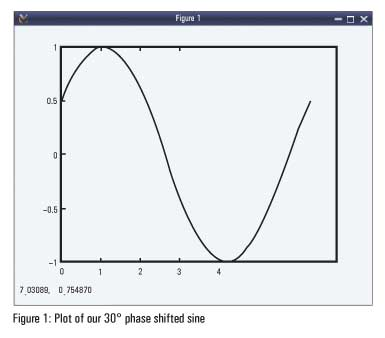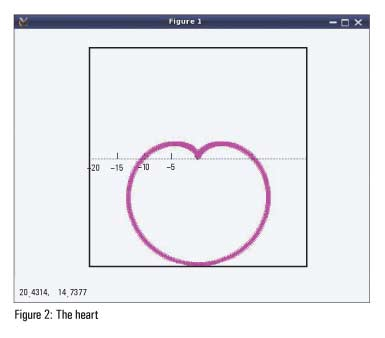# Mathematics Made Easy With Minimal Octave

0
4656This fifth article in the mathematical journey through open source introduces Octave, a non-programmer’s way of doing mathematics.

Octave sounds like a musical note, but in reality it is the name of its author’s Chemical Engineering professor, who was known for his ‘back-of-the-envelope calculations’.

All of bench calculator, and more!
All the operations of the bench calculator (bc) are just a subset of octave. So, there’s no point in reinventing the wheel. Whatever operations can be done in bc–arithmetical, logical, relational and conditional–can also be done with equal ease in octave. And like bc, it can be used as a mathematical programming language. So, why did we waste time learning bc? We might as well have come directly to octave. Well, there’s one difference–precision. You can’t get that in octave. If you need it, you would have to go back to bc. With octave, let’s start with N-dimensions, or in other words, vectors and matrices.

Getting started
Commands: Typing ‘octave’ on the shell brings up the octave’s interactive shell. Type ‘quit’ or ‘Control-D’ to exit. The interactive shell starts with a welcome message. As in bc, use the option -q for it not to show. Additionally, use the -f option for it not to pick up any local start-up scripts (if any). So, for our examples, the command would be ‘octave -qf’ to start octave with an interactive shell.
Prompts and results: The input prompt is typically denoted by ‘octave:X>’, where X is just a number showing the command count. Valid results are typically shown with ‘<variable> = ‘, or ‘ans = ‘ or ‘=> ‘. The command count is incremented for the next input. Errors cause error messages to be printed and then octave brings back the input prompt without incrementing the command count. Putting a semicolon (;) at the end of a statement suppresses the result (return value) to be displayed.Comments could start with # or %. For block comments, #{ … }# or %{ … }% can be used. Detailed help on a particular topic can be obtained using ‘help <topic>’ and the complete documentation can be accessed using ‘doc’ on the octave shell.

```\$ octave -qf
octave:1> # This is a comment
octave:1> pi # Built-in constant
ans =  3.1416
octave:2> e # Built-in constant again
ans =  2.7183
octave:3> i # Same as j – the imaginary number
ans =  0 + 1i
octave:4> x = 3^4 + 2*30;
octave:5> x
x =  141
octave:6> y
error: `y' undefined near line 6 column 1
octave:6> doc # Complete doc; Press 'q' to come back
octave:7> help plot # Help on plot
octave:8> A = [1 2 3; 4 5 6; 7 8 9] # 3x3 matrix
A =

1   2   3
4   5   6
7   8   9

octave:9> quit```

Matrices with a heart

We have already seen matrix creation. They can also be created through multiple lines. Octave continues waiting for further inputs by just prompting ‘>’. Check below for how to create the 3×3 magic square matrix, followed by various other interesting operations:

```\$ octave -qf
octave:1> M = [ # 3x3 magic square
> 8 1 6
> 3 5 7
> 4 9 2
> ]
M =

8   1   6
3   5   7
4   9   2

octave:2> B = rand(3, 4); # 3x4 matrix w/ randoms in [0 1]
octave:3> B
B =

0.068885   0.885998   0.542059   0.797678
0.652617   0.904360   0.036035   0.737404
0.043852   0.579838   0.709194   0.053118

octave:4> B' # Transpose of B
ans =

0.068885   0.652617   0.043852
0.885998   0.904360   0.579838
0.542059   0.036035   0.709194
0.797678   0.737404   0.053118

octave:5> A = inv(M) # Inverse of M
A =

0.147222  -0.144444   0.063889
-0.061111   0.022222   0.105556
-0.019444   0.188889  -0.102778

octave:6> M * A # Should be identity, at least approx.
ans =

1.00000   0.00000  -0.00000
-0.00000   1.00000   0.00000
0.00000   0.00000   1.00000

octave:7> function rv = psine(x) # Our phase shifted sine
>
> rv = sin(x + pi / 6);
>
>  endfunction
octave:8> x = linspace(0, 2*pi, 400); # 400 pts from 0 to 2*pi
octave:9> plot(x, psine(x)) # Our function's plot
octave:10> polar(x, 10 * (1 - sin(x)), 'm*') # bonus Heart
octave:11> quit```

Figure 1 shows the plot window that pops up in response to command # 9 – plot and Figure 2 is the bonus magenta heart of stars (*) from polar coordinates draw command # 10 – polar.

What next?
With a ready-to-go level of introduction to octave, we are all set to explore it the fun way. What fun, you may well ask. That’s left to your imagination. And as we move on, you could take up one or more fun challenge(s) and try to solve them using octave.